## Standard Deviation

The standard deviation is defined as the Square Root of the Variance,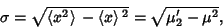(1)

whereis the Mean and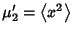is the second Moment about 0. The variance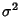is equal to the second Moment about the Mean,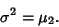(2)

The square root of the Sample Variance is the sample'' standard deviation,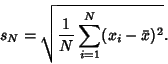(3)

It is a Biased Estimator of the population standard deviation. As unbiased Estimator is given by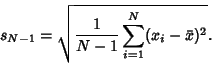(4)

Physical scientists often use the term Root-Mean-Square as a synonym for standard deviation when they refer to the Square Root of the mean squared deviation of a signal from a given baseline or fit.